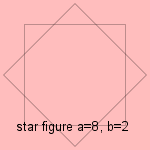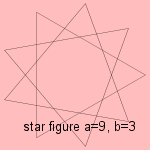# star figure

## line

last updated: 2005-11-04

The star figure is a variation on the star polygon.
Star figure or star polygon is sometimes called a polygram.

Given a regularly placed points on a circumference. By connecting each bth point, a star figure (a,b) is formed when a and b are not relatively prime.
The curve consists of two or more (simple) polygons.

Some examples:

•a=6, b=2: the hexagram, also named star of David or Jews' star (a=6, b=2), named to the Jewish king David, is the symbol of the Jews.
The curve is a combination of two triangles.

•a=8, b=2: the star of Lakshmi.
It represents Ashthalakshmi, the eight forms of the Hindu goddess of fortune and prosperity named Lakshmi.
The curve is a combination of two squares.

•a=9, b=3: nonagram or star of Goliath
The curve is composed of three equilateral triangles rotated at angles 0, 40 and 80 degrees.